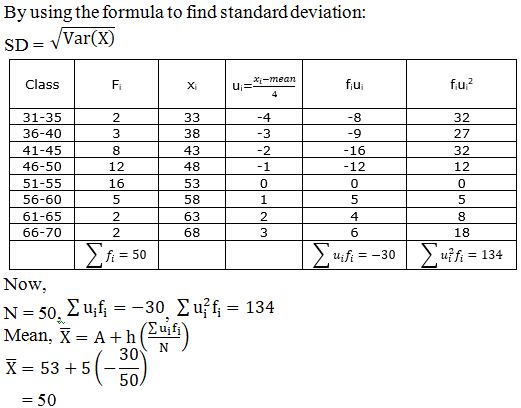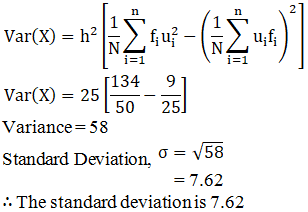# RD Sharma Solutions for Class 11 Chapter 32 - Statistics Exercise 32.6

Here, we shall deal with variance of a grouped or continuous frequency distribution, which are explained using examples. Numerous solved examples are provided here in this exercise to help students understand the method of solving problems using shortcut techniques. Students can boost their exam preparation by solving the textbook problems, using the solutions created by the subject experts. These solutions are explained in simple language, keeping in mind the intelligence quotient of students. Students can access RD Sharma Class 11 Maths Solutions and download the pdf, from the below mentioned links.

## Download the pdf of RD Sharma Solutions for Class 11 Maths Exercise 32.6 Chapter 32 – Statistics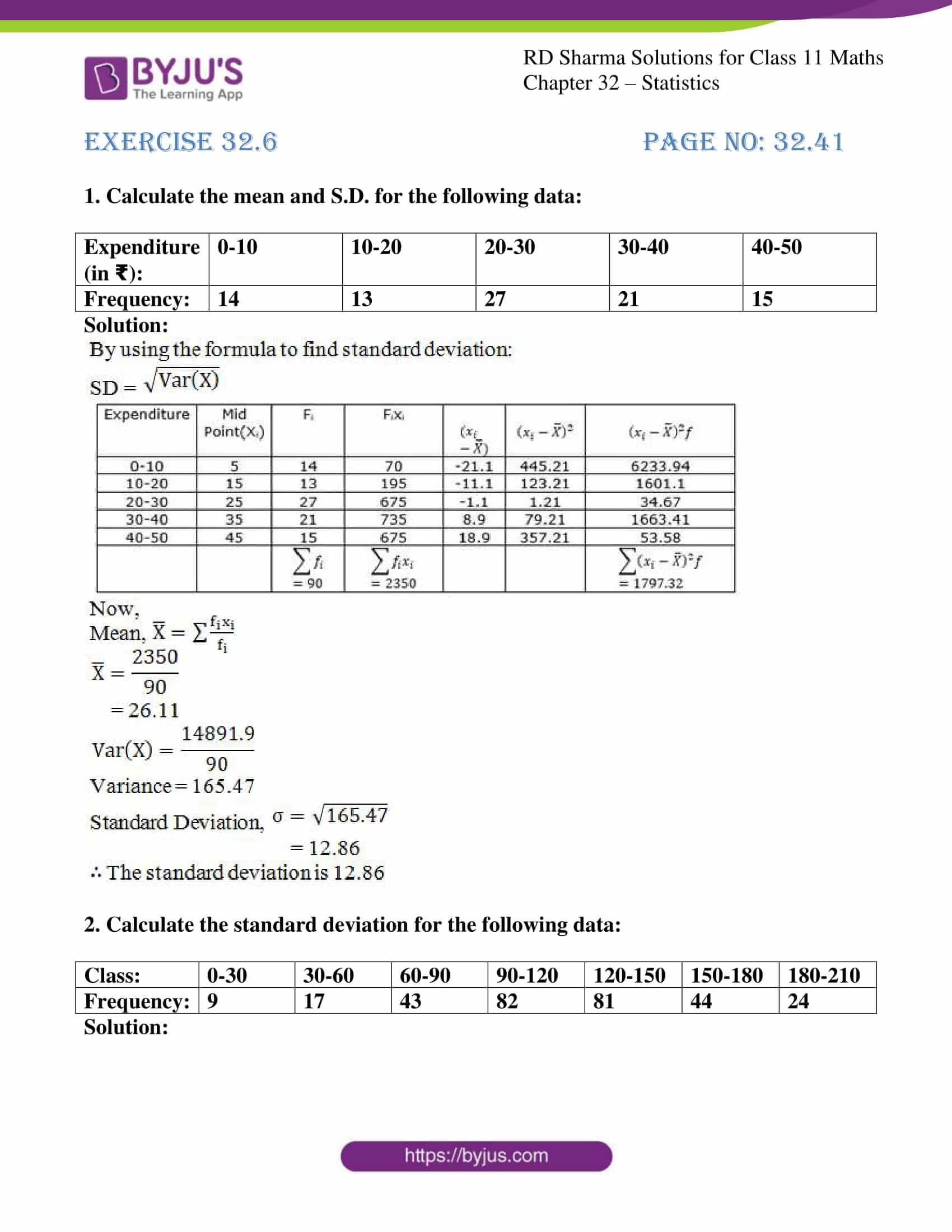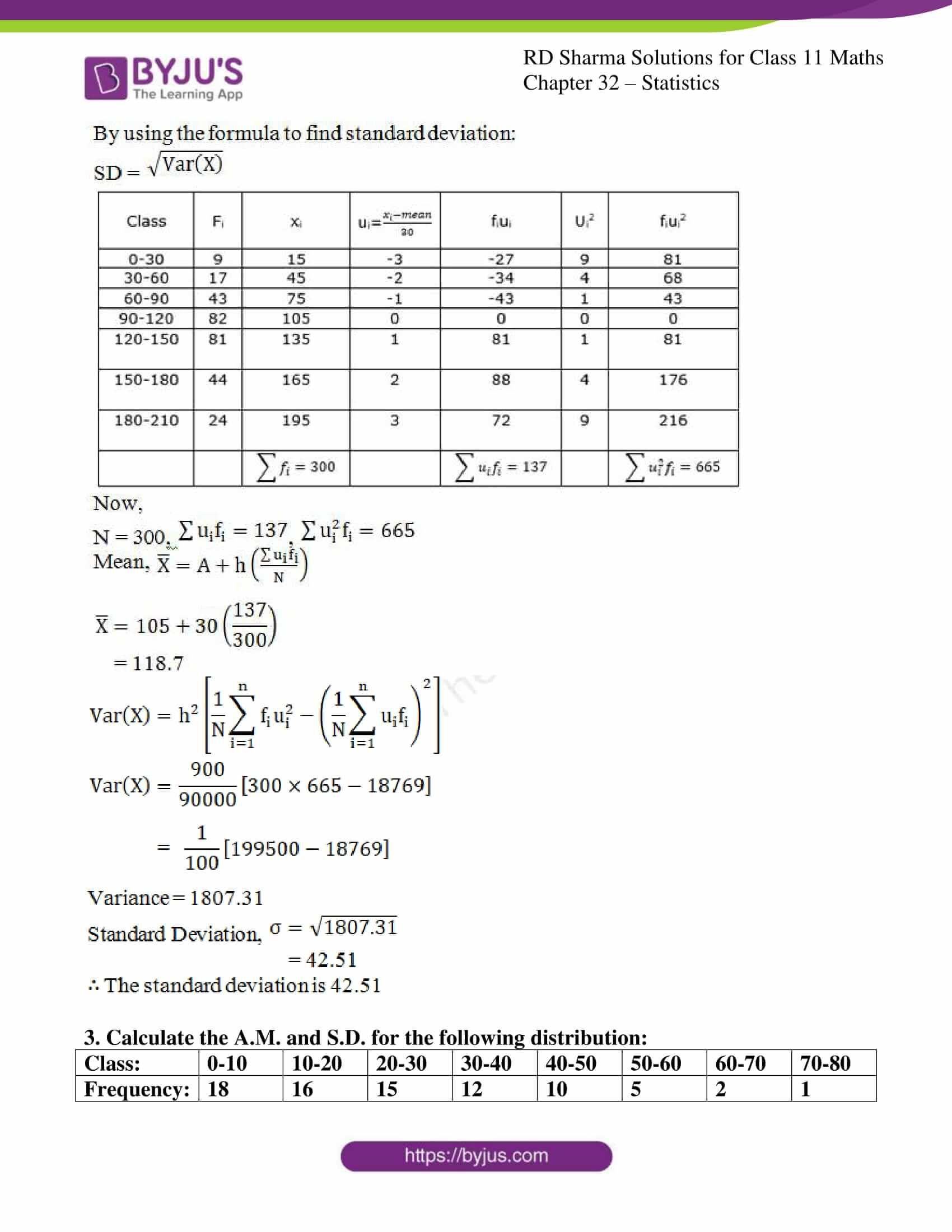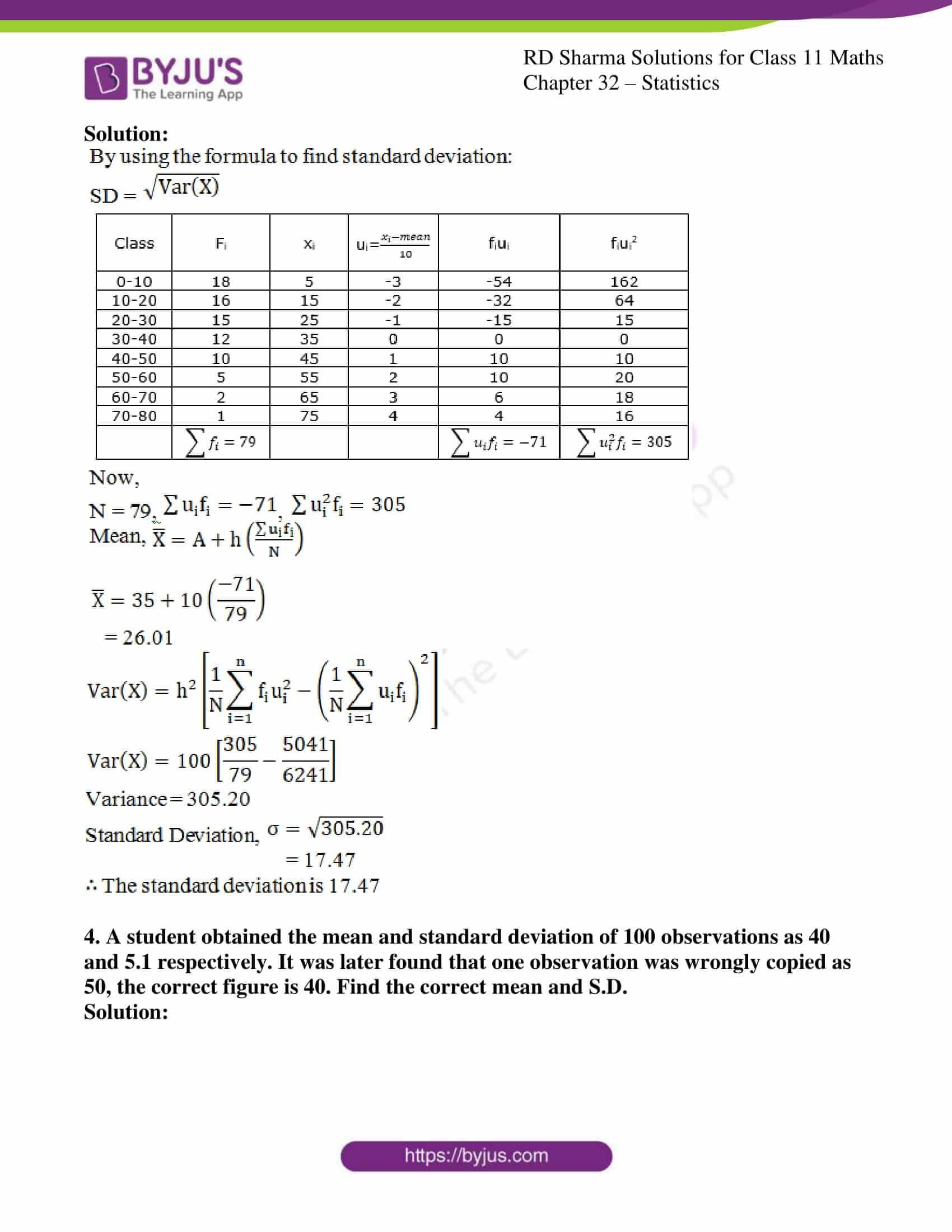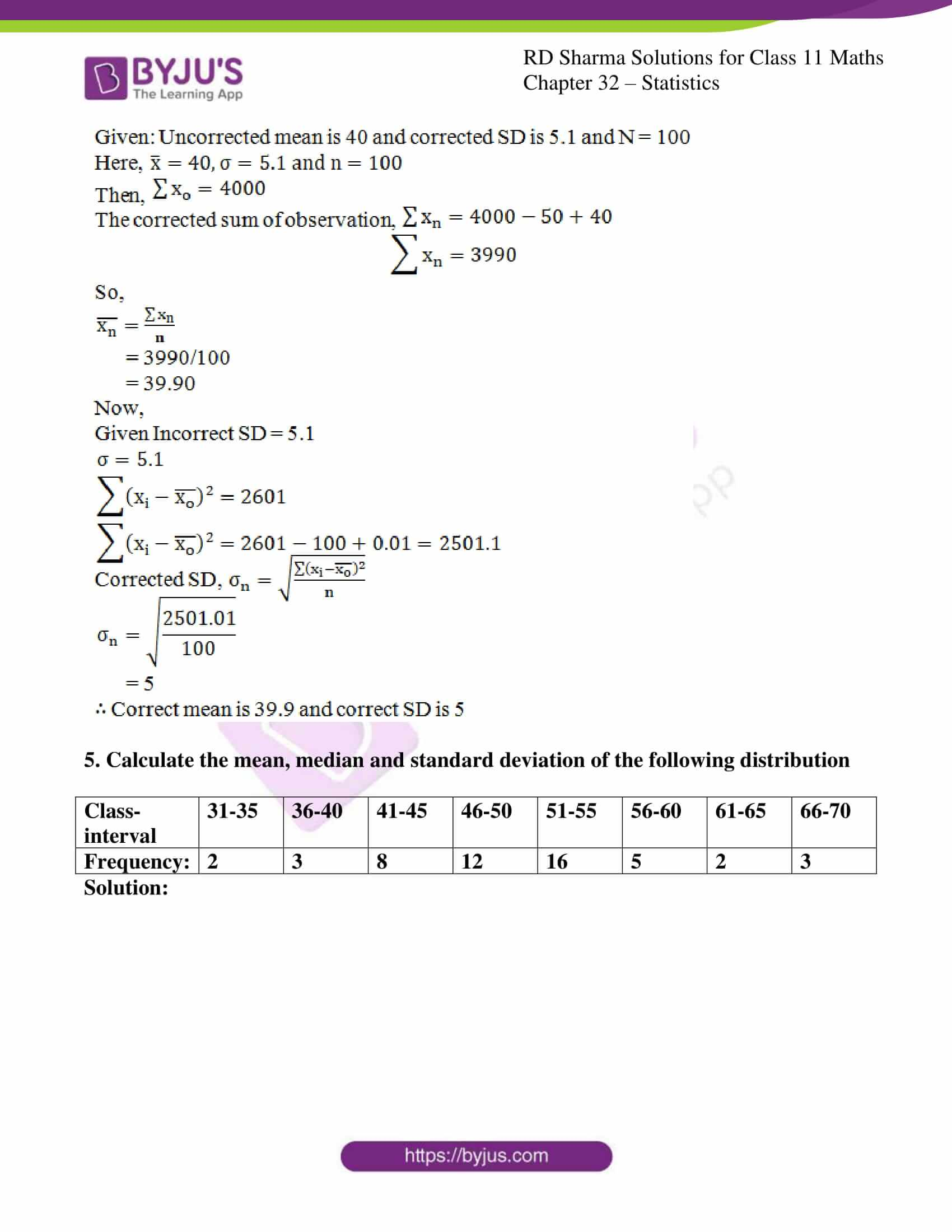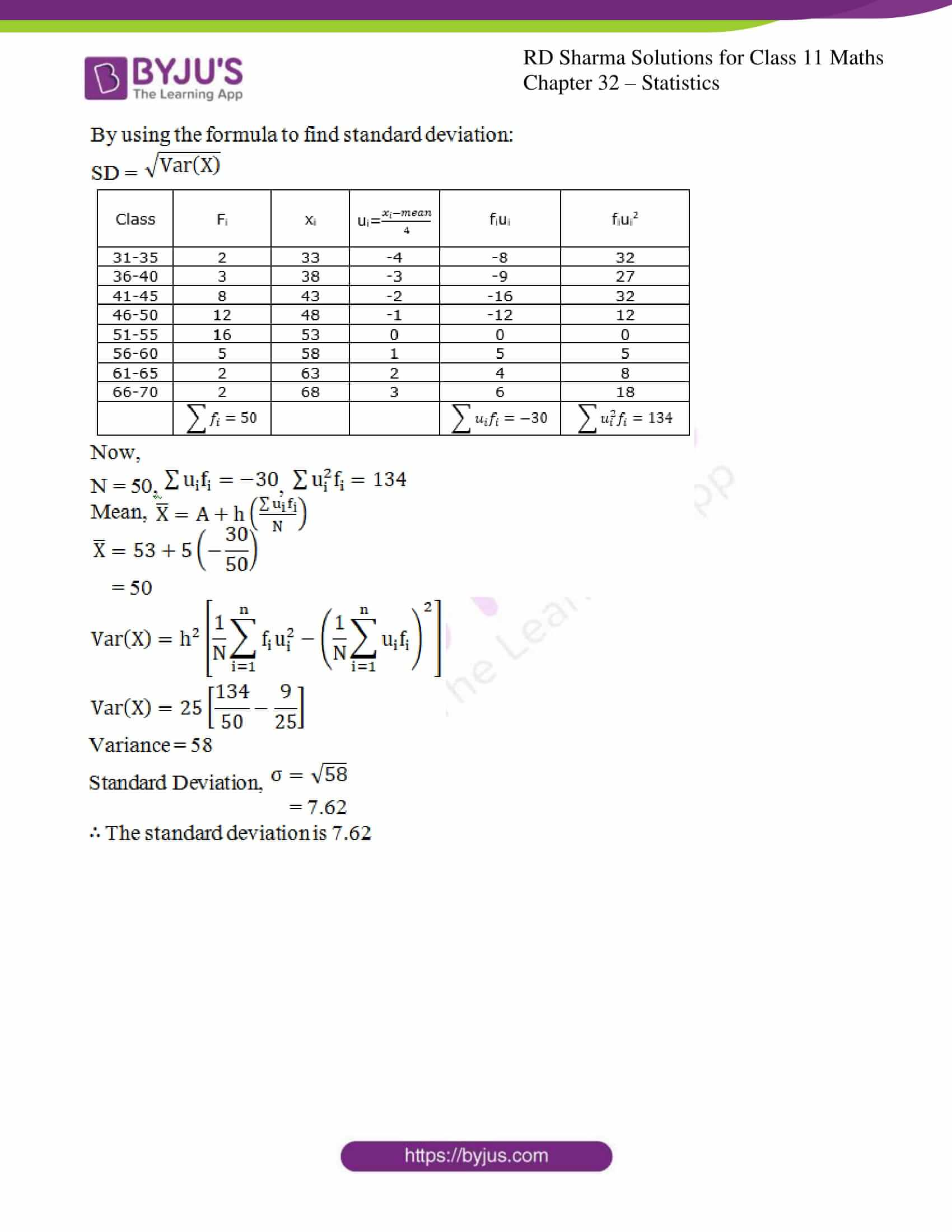### Access answers to RD Sharma Solutions for Class 11 Maths Exercise 32.6 Chapter 32 – Statistics

#### EXERCISE 32.6 PAGE NO: 32.41

1. Calculate the mean and S.D. for the following data:

 Expenditure (in ₹): 0-10 10-20 20-30 30-40 40-50 Frequency: 14 13 27 21 15

Solution: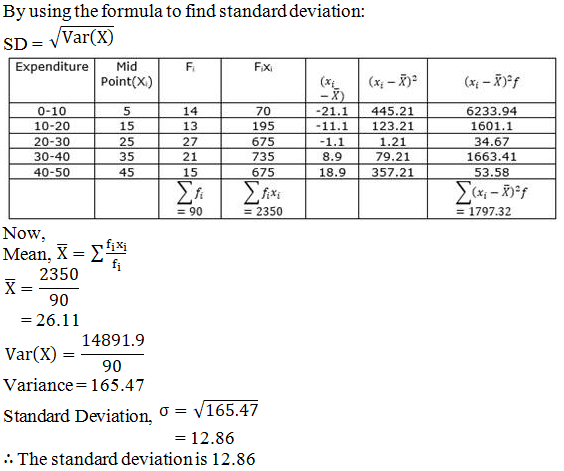2. Calculate the standard deviation for the following data:

 Class: 0-30 30-60 60-90 90-120 120-150 150-180 180-210 Frequency: 9 17 43 82 81 44 24

Solution: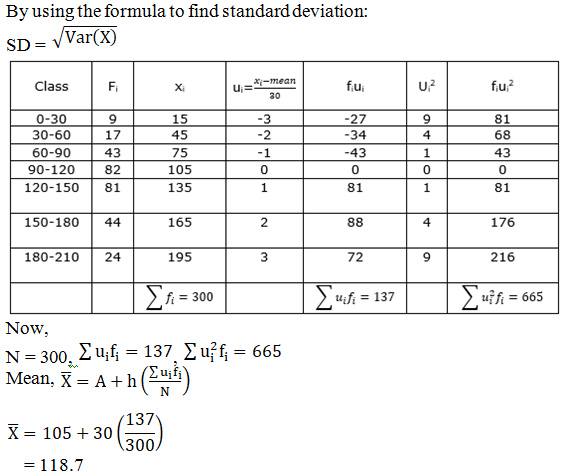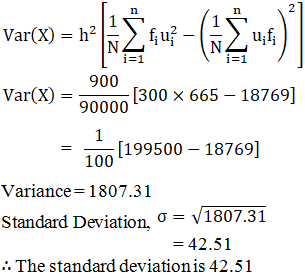3. Calculate the A.M. and S.D. for the following distribution:

 Class: 0-10 10-20 20-30 30-40 40-50 50-60 60-70 70-80 Frequency: 18 16 15 12 10 5 2 1

Solution: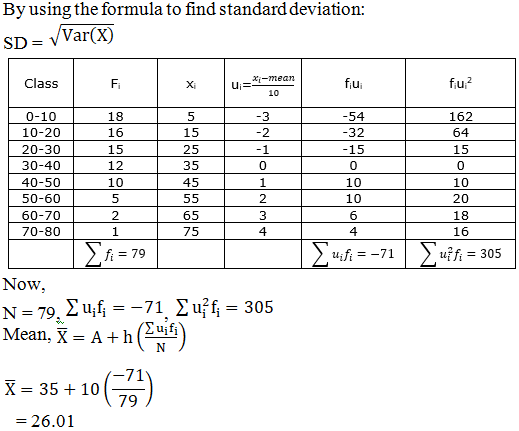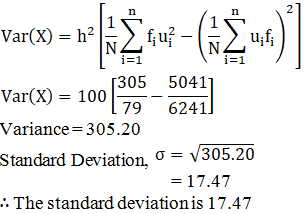4. A student obtained the mean and standard deviation of 100 observations as 40 and 5.1 respectively. It was later found that one observation was wrongly copied as 50, the correct figure is 40. Find the correct mean and S.D.

Solution: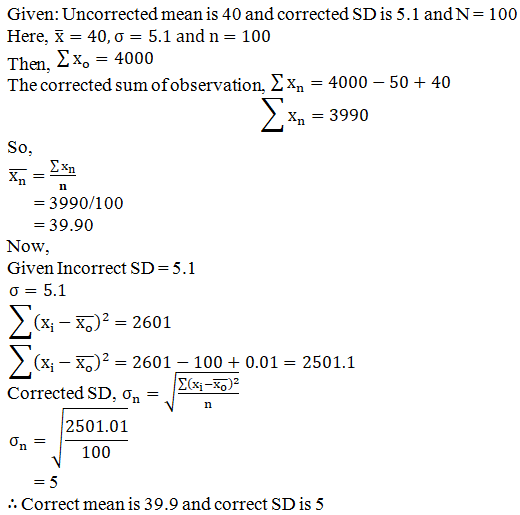5. Calculate the mean, median and standard deviation of the following distribution

 Class-interval 31-35 36-40 41-45 46-50 51-55 56-60 61-65 66-70 Frequency: 2 3 8 12 16 5 2 3

Solution: